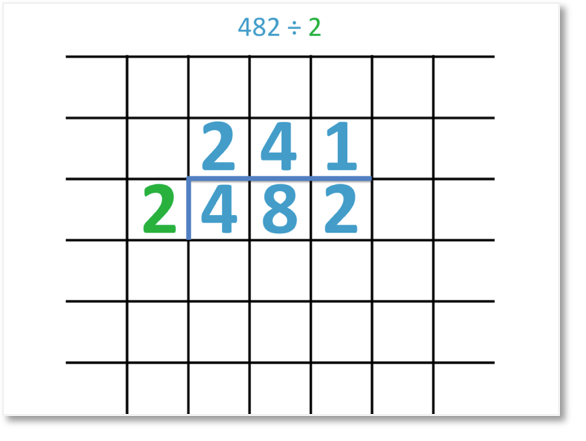# Division by Grouping

Division by Grouping• Division by grouping is a strategy used to introduce the concept of division.
• Here we can draw 10 dots or use 10 counters.
• Collect this amount into groups of the number we are dividing by.
• We are dividing by 5, so we collect the counters into groups of 5.
• We have 2 groups and so, the answer is 2.
• The answer to a division tells us how many groups of a number can be made.
• Two groups of five can be made from 10 counters.

We make groups of the number that we are dividing by.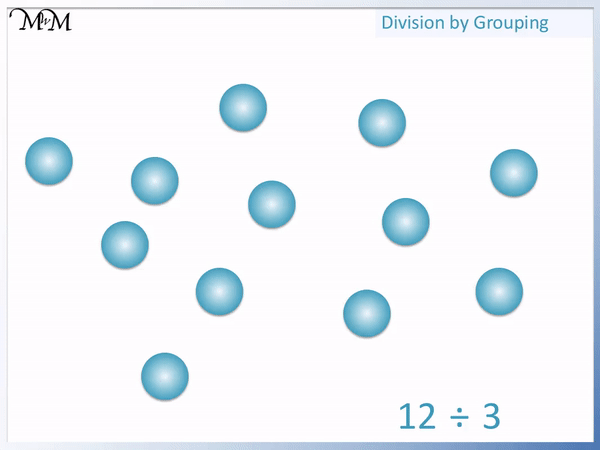• We start with 12 counters or we can draw 12 dots.
• We are dividing by 3 so we collect them into groups of 3.
• We can count 4 groups in total.
• 12 ÷ 3 = 4.
• This means that 4 groups of 3 can be made from 12 counters.Supporting Lessons# Division by Grouping

## What is Division by Grouping?

Division by grouping is a strategy used to introduce the concept of division. It involves collecting an amount into equal groups and counting how many groups can be made.

The amount in each group is the number being divided by and the number of groups that can be made is the answer to the division.

For example, here is the division of 6 ÷ 2.

We start with the amount being divided, which is 6. We have 6 counters.We are dividing 6 by 2 because there is a 2 after the division sign in 6 ÷ 2.

The answer to this division tells us how many times 2 goes into 6.

We can also think of this as, “How many groups of 2 can be made from 6 counters?”.

We collect the 6 counters into groups of 2 as shown below.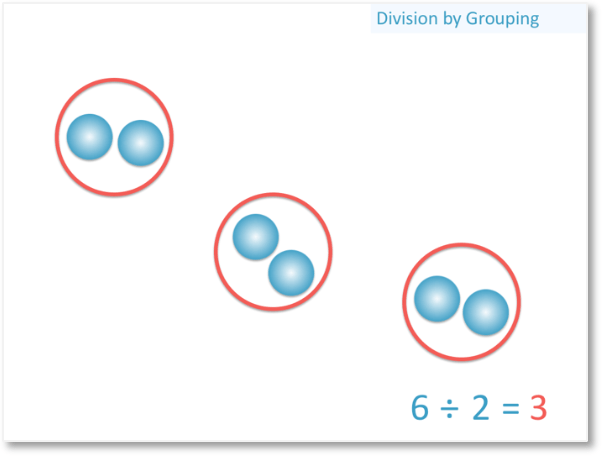We have drawn circles around the counters so that there are 2 counters in each group.

We have 3 circled groups, which means that 3 groups of 2 can be made.

Our answer to the division is 3.The answer to a division is the number of equal groups that can be made.

We have 3 groups and so, 3 is the answer to the division 6 ÷ 2 = 3.

This also tells us that two goes into six three times.

## How to Divide with Equal Groups

To divide with equal groups use the following steps:

1. Draw the same number of dots as the amount being divided.
2. Count dots until you have the number being divided by.
3. Draw a circle around these dots.
4. Repeat steps 2 & 3 until all of the dots are grouped.
5. Count the number of groups you have.
6. The number of groups is the answer to the division.

For example we have 12 ÷ 3.

We draw 12 dots because 12 is the number in our division that is being divided. 12 comes first in the division.We are dividing by 3 because three comes after the division sign in 12 ÷ 3.

We will count groups of 3 dots and draw a circle around them.We can keep grouping the dots into groups of 3 until we have 4 groups in total.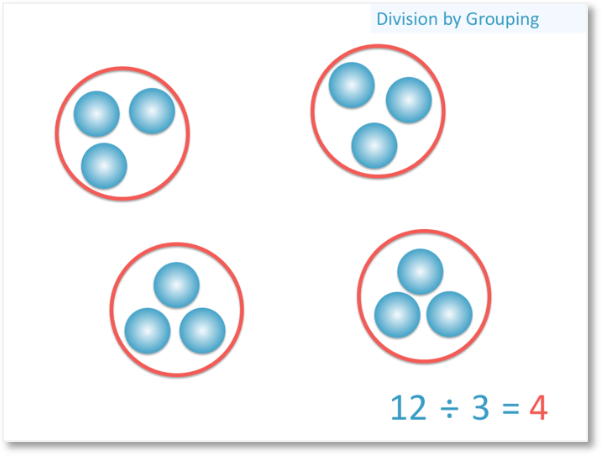We have 4 groups in total and so, the answer to the division is 4.

12 ÷ 3 = 4

This means that we can make 4 groups of 3 dots from 12 dots.

## How to Teach Division by Grouping

When teaching division by grouping we start by introducing division as a physical model. We can use counters to do this or we can draw dots to represent the number.

The method of division by grouping helps to reinforce the meaning behind division and the process of sharing into equal groups helps some people to retain the method.

Division by grouping is not a method that would be recommended for the long term but it can be used to support students who are still learning their times tables and division facts.

Here is 10 ÷ 5.

We can draw 10 dots if learning this method on paper but counters can be an easier and more engaging way to introduce it.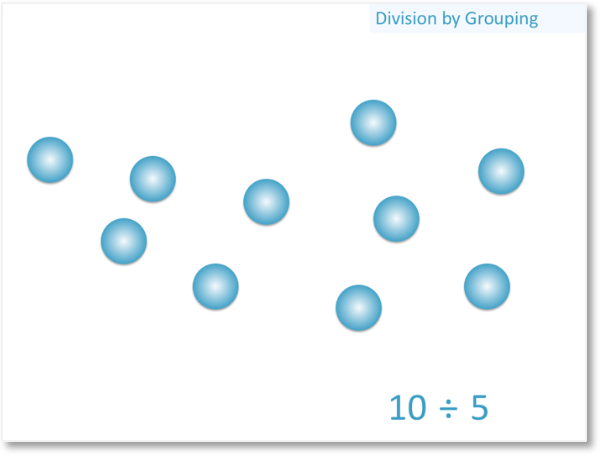We can put a line through each dot as we count them, or if we are using counters, we can pick them up as we count them.

We count groups of five.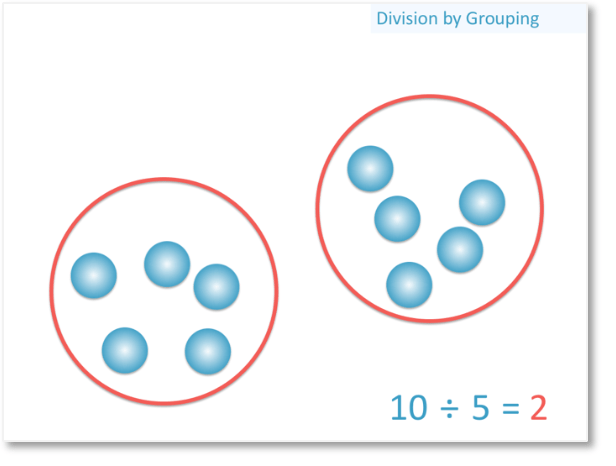We can draw around the dots if we drew them and this can be a simple written method that some children will use to calculate division if they are still remembering their division facts.

If using counters, we can pick up the counters and put them into pots or piles.The number of circles, pots or piles that we have is the answer.

We have 2 equal groups so 10 ÷ 5 = 2.

Division by grouping is a worthwhile method to support understanding of the concept of division but we want to encourage children to move onto memorising the division facts shortly after this.

Division facts are like times tables in reverse. If we know that 2 × 5 = 10, then we know the division fact that 10 ÷ 5 = 2.

As mathematical methods become more complex, it is good to know division facts fairly quickly, so that less time and effort is required to concentrate on the division and more time can be devoted to learning the new procedures.Now try our lesson on Short Division without Remainders where we introduce the short division method.